Author: Oscar Cronquist Article last updated on February 01, 2019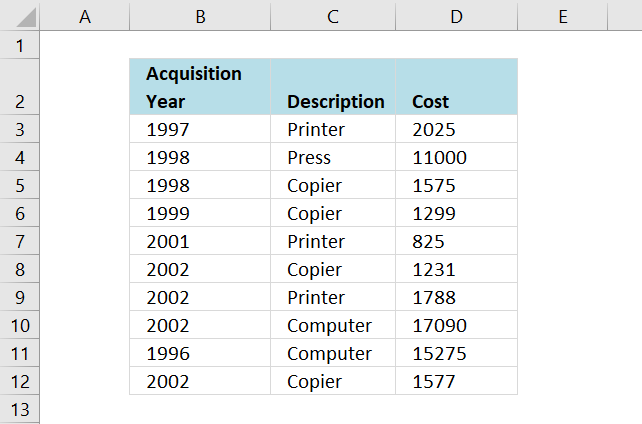I will in this blog post demonstrate a formula that extracts common records (shared records) from two data sets in Excel. I have demonstrated how to compare two columns and today I want to show you how to filter records that exists in both tables.

You can also use conditional formatting to highlight shared records. If you are looking for records that exist only in one out of two tables then read this article: Filter records occurring in only one table

The first data set is in worksheet: List 1, see image above. The second data set is in worksheet: List 2, see image below. These two data sets have three columns each that I want to compare. One column has a different header name.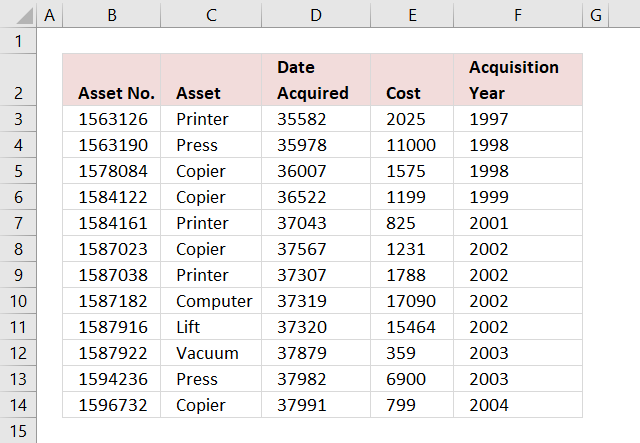The columns I am going to compare are these:

• List 1 : Description - List 2 : Asset
• List 1 : Cost - List 2 : Cost
• List 1 : Acquisition year - List 2 : Acquisition year

The following picture shows the columns I am going to compare. Keep in mind that records must be exactly the same in both data tables to be filtered, except that letter case may differ.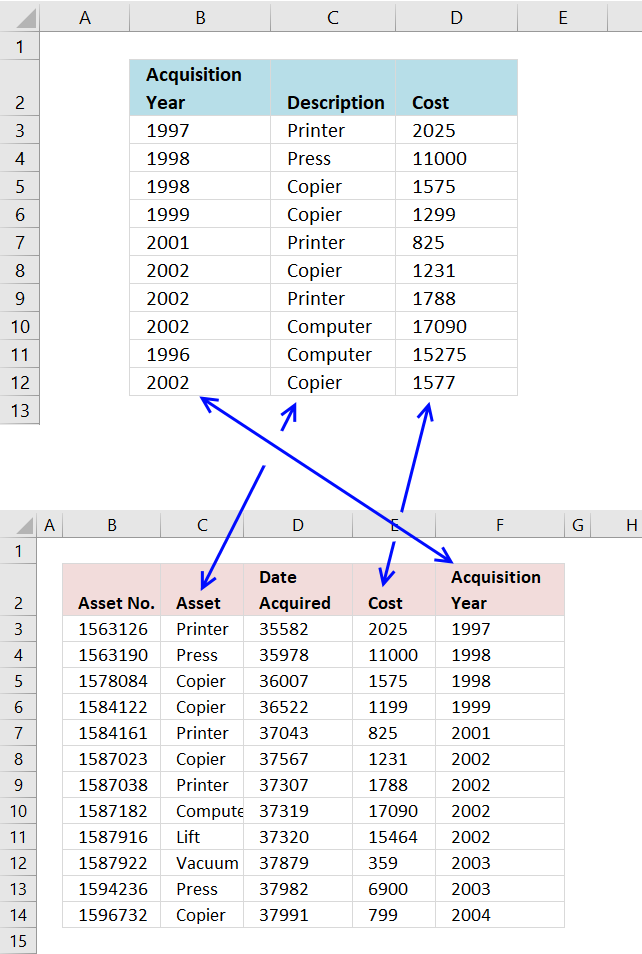COUNTIFS is the core in the formula I will construct, it is here all the comparisons will take place. It is important to understand what is going on so you can use this technique to create your own powerful array formulas.

The COUNTIFS function may have up to 255 arguments leaving you to compare up to 127 columns, however, if you have that many columns to compare perhaps a UDF (User defined function) is a better option. Array formulas are often quite slow dealing with lots of data.

COUNTIFS(criteria_range1,criteria1, criteria_range2, criteria2...)
Counts the number of cells specified by a given set of conditions or criteria

Here are the cell ranges that I am going to use in the COUNTIFS function:

List 2 - Aquisition year - 'List 2'!\$F\$3:\$F\$14
List 1 - Aquisition year - 'List 1'!\$B\$3:\$B\$12

List 2 - Asset - 'List 2'!\$C\$3:\$C\$14
List 1 - Description - 'List 1'!\$C\$3:\$C\$12

List 2 - Cost - 'List 2'!\$E\$3:\$E\$14
List 1 - Cost - 'List 1'!\$D\$3:\$D\$12

COUNTIFS('List 2'!\$F\$3:\$F\$14,'List 1'!\$B\$3:\$B\$12,'List 2'!\$C\$3:\$C\$14,'List 1'!\$C\$3:\$C\$12,'List 2'!\$E\$3:\$E\$14,'List 1'!\$D\$3:\$D\$12)

Is it important to begin with the second data set in the first argument? No, you can begin with the first data set if you like, remember to change cell reference in the INDEX function so you fetch the right values.

With the COUNTIFS function complete we can now construct the array formula.

### Array formula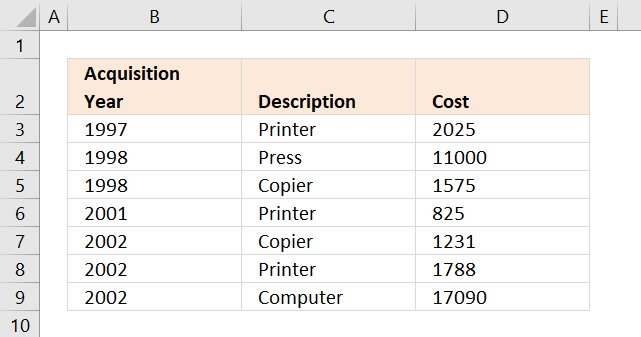The image above shows the third worksheet named: Common records

Array formula in cell B3:

=INDEX('List 1'!\$B\$3:\$D\$12,SMALL(IF(COUNTIFS('List 2'!\$F\$3:\$F\$14,'List 1'!\$B\$3:\$B\$12,'List 2'!\$C\$3:\$C\$14,'List 1'!\$C\$3:\$C\$12,'List 2'!\$E\$3:\$E\$14,'List 1'!\$D\$3:\$D\$12),MATCH(ROW('List 1'!\$B\$3:\$B\$12),ROW('List 1'!\$B\$3:\$B\$12)),""),ROWS(\$A\$1:A1)),COLUMNS(\$A\$1:A1))

### How to create an array formula

1. Select cell B3
2. Press with left mouse button on in formula bar3. Copy the array formula above and paste to formula bar
4. Press and hold Ctrl + Shift simultaneously
5. Press Enter
6. Release all keys

You can check using the formula bar that you did above steps right, Excel tells you if a cell contains an array formula by surrounding the formula with a beginning and ending curly brackets, like this: {=array_formula}.

Don't enter these characters yourself they show up automatically if you did above steps correctly.

Copy cell B3 and paste it to the right as far as needed. Copy cell B3:D3 and paste down as far as needed.

### Explaining the array formula in cell B3

You can easily examine a formula (or array formula) that you don't understand, select the cell containing the formula. Go to tab "Formulas", press with left mouse button on "Evaluate Formula".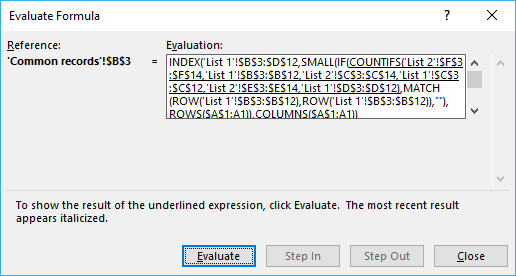The "Evaluate" button above lets you go to the next "calculation" step.

Step 1 - Find common records

COUNTIFS('List 2'!\$F\$3:\$F\$14,'List 1'!\$B\$3:\$B\$12,'List 2'!\$C\$3:\$C\$14,'List 1'!\$C\$3:\$C\$12,'List 2'!\$E\$3:\$E\$14,'List 1'!\$D\$3:\$D\$12)

becomes

COUNTIFS({1997, 1998, 1998, 1999, 2001, 2002, 2002, 2002, 2002, 2003, 2003, 2004},{1997, 1998, 1998, 1999, 2001, 2002, 2002, 2002, 1996, 2002}, {Printer, Press, Copier, Copier, Printer, Copier, Printer, Computer, Lift, Vacuum, Press, Copier},{Printer, Press, Copier, Copier, Printer, Copier, Printer, Computer, Computer, Copier},{2025, 11000, 1575, 1199, 825, 1231, 1788, 17090, 15464, 359, 6900, 799},{2025, 11000, 1575, 1299, 825, 1231, 1788, 17090, 15275, 1577})

and returns this array: {1, 1, 1, 0, 1, 1, 1, 1, 0, 0}

The array is shown in column F below, the first 1 in the array means that the three corresponding values on row 3 (B3, C3 and D3) have a match somewhere on the other data table, on the same row.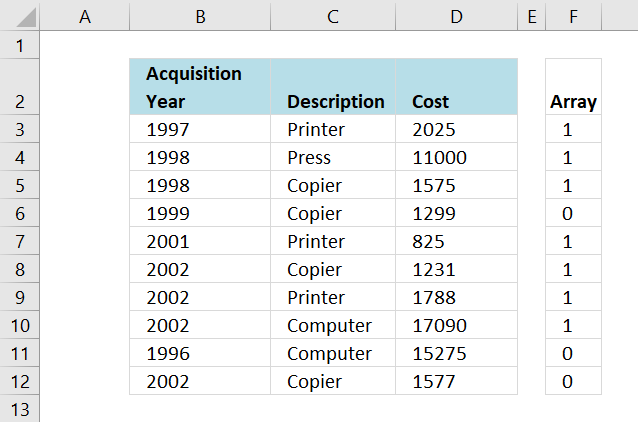Recommended articles

How to use the COUNTIFS function
Checks multiple conditions against the same number of cell ranges and counts how many times all criteria are met.

Step 2 - Return corresponding relative row numbers

IF(COUNTIFS('List 2'!\$F\$3:\$F\$14,'List 1'!\$B\$3:\$B\$12,'List 2'!\$C\$3:\$C\$14,'List 1'!\$C\$3:\$C\$12,'List 2'!\$E\$3:\$E\$14,'List 1'!\$D\$3:\$D\$12),MATCH(ROW('List 1'!\$B\$3:\$B\$12),ROW('List 1'!\$B\$3:\$B\$12)),"")

becomes

IF({1, 1, 1, 0, 1, 1, 1, 1, 0, 0}, MATCH(ROW('List 1'!\$B\$3:\$B\$12),ROW('List 1'!\$B\$3:\$B\$12)),"")

becomes

IF({TRUE, TRUE, TRUE, FALSE, TRUE, TRUE, TRUE, TRUE, FALSE, FALSE}, {1, 2, 3, 4, 5, 6, 7, 8, 9},"")

and returns {1,2,3,"",5,6,7,"",""}

The image below shows you relative row numbers for records that exist on the other data table.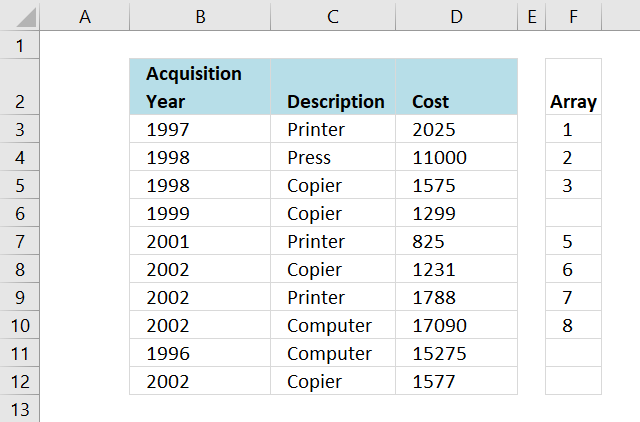Now it is really easy for the INDEX function to fetch the values it need, first I need to make this array formula return a single number. The SMALL function helps me with that.

Recommended articles

How to use the IF function
Checks if a logical expression is met. Returns a specific value if TRUE and another specific value if FALSE.

Step 3 - Return the k-th smallest value

I don't want the SMALL function to extract the same value in every cell, I want it to change so a new row number is extracted in the cell below. This is repeated in every new cell below until all values have been extracted.

SMALL({1,2,3,"",5,6,7,"",""}, ROWS(\$A\$1:A1))

becomes

SMALL({1,2,3,"",5,6,7,"",""}, 1)

and returns 1.

The ROWS function return the number of rows a certain cell range has. If that cell range expands every time I copy the formula to new cells below, the ROWS function will return the old number + 1.

In cell B3 ROWS(\$A\$1:A1) returns 1. In cell B4 it changes to ROWS(\$A\$1:A2) and returns 2. Read more about absolute and relative cell references here.

Why use the ROWS function and not ROW? If you insert new rows above the formula, the ROW function will return the wrong value because the relative cell reference changed. It will change in the ROWS function as well but so will also the absolute cell reference.

Recommended articles

How to use the SMALL function
The SMALL function lets you extract a number in a cell range based on how small it is compared to the other numbers in the group.

Step 4 - Return a value of the cell at the intersection of a particular row and column

INDEX('List 1'!\$B\$3:\$D\$12,SMALL(IF(COUNTIFS('List 2'!\$F\$3:\$F\$14,'List 1'!\$B\$3:\$B\$12,'List 2'!\$C\$3:\$C\$14,'List 1'!\$C\$3:\$C\$12,'List 2'!\$E\$3:\$E\$14,'List 1'!\$D\$3:\$D\$12),MATCH(ROW('List 1'!\$B\$3:\$B\$12),ROW('List 1'!\$B\$3:\$B\$12)),""),ROWS(\$A\$1:A1)),COLUMNS(\$A\$1:A1))

becomes

=INDEX('List 1'!\$A\$2:\$C\$11, 1, COLUMNS(\$A\$1:A1))

becomes

=INDEX('List 1'!\$A\$2:\$C\$11, 1, 1)

and returns 1997 in cell B3.Recommended articles

How to use the INDEX function
Gets a value in a specific cell range based on a row and column number.

### Get the Excel fileCompare-lists.xlsx